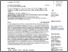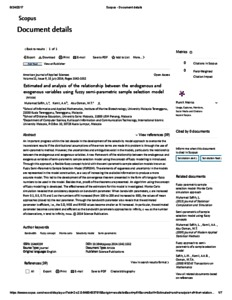Estimated and analysis of the relationship between the endogenous and exogenous variables using fuzzy semi-paranetric sample selection model

MuhamadSafiih, L and Kamil, A.A. and Abu Osman, M.T. (2014) Estimated and analysis of the relationship between the endogenous and exogenous variables using fuzzy semi-paranetric sample selection model. American Journal of Applied Sciences, 11 (9). pp. 1542-1552. ISSN 1554-3641(O), 1546-9239 (P)Preview
PDF - Published VersionPreview
PDF

Abstract

An important progress within the last decade in the development of the selectivity model approach to overcome the inconsistent results if the distributional assumptions of the errors terms are made this problem is through the use of semi-parametric method. However, the uncertainties and ambiguities exist in the models, particularly the relationship between the endogenous and exogenous variables. A new framework of the relationship between the endogenous and exogenous variables of semi-parametric sample selection model using the concept of fuzzy modelling is introduced. Through this approach, a flexible fuzzy concept hybrid with the semi-parametric sample selection models known as Fuzzy Semi-Parametric Sample Selection Model (FSPSSM). The elements of vagueness and uncertainty in the models are represented in the model construction, as a way of increasing the available information to produce a more accurate model. This led to the development of the convergence theorem presented in the form of triangular fuzzy numbers to be used in the model. Besides that, proofs of the theorems are presented. An algorithm using the concept of fuzzy modelling is developed. The effectiveness of the estimators for this model is investigated. Monte Carlo simulation revealed that consistency depends on bandwidth parameter. When bandwidth parameters, c are increased from 0.1, 0.5, 0.75 and 1 as the numbers of N increased (from 100 to 200 and increased to 500), the values of mean approaches (closed to) the real parameter. Through the bandwidth parameter also reveals that the estimated parameter is efficient, i.e., the S.D, MSE and RMSE values become smaller as N increased. In particular, the estimated parameter becomes consistent and efficient as the bandwidth parameters approaches to infinity, c®¥ as the number of observations, n tend to infinity, n®¥. Keywords: Selectivity Model, Semi-Parametric, Fuzzy Concept, Bandwidth, Monte Carlo

Item Type: Article (Journal) 5996/40312 Selectivity Model, Semi-Parametric, Fuzzy Concept, Bandwidth, Monte Carlo Q Science > QA Mathematics Kulliyyah of Information and Communication Technology > Department of Computer ScienceKulliyyah of Information and Communication Technology > Department of Computer Science Prof Dr ABU OSMAN MD TAP 27 Dec 2014 16:51 24 Aug 2017 15:17 http://irep.iium.edu.my/id/eprint/40312View Item